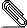# [NTG-context] Kerning/italic correction problem with TeX Gyre Bonum font

Hi Hans and others!

I don't know if this is a problem with the font itself or if it is ConTeXt.
In any case, the test file below gives strange output (attached). In
particular the space/kerning after $f$ seems to be off.

Ulrike Fischer reminded me in the StackExchange chat that not so long time
ago there was some similar issue with \mathcal P discussed on this list. Is
it the same problem? (The input $f \tracinglostchars=0 \Uchar"EEEFF$)
indeed makes it look better between the $f$ and the parenthesis.)

/Mikael

%%%
\setupbodyfont[bonum]

\starttext
\startTEXpage[offset=3bp]
(Look at this $f$) and this $f$? (see also $k$?, $x$? and $z$?)

There might be more problems (like between $t$ and the parenthesis in
$e^t$) is too small.

The space between $dx$ and the bar is too small:
\startformula
\Bigl|\int_0^\pi\sin x\,dx\Bigr|,
\stopformula
Look at the $f$ and the bar:
\startformula
\Bigl|\int f\Bigr|
\stopformula
The $x$ seems to be outside the fraction:
\startformula
\frac{a^2}{1-x}
\stopformula
We add another one (in fact two) including $f$:
\startformula
f\Bigl(\frac{a+b}{2}\Bigr)
\stopformula

Also look at $\xi_0$ and $\xi_1$, the subscripts are too far away from
$\xi$. Compare with $f_0$ and $f_1$, $g_0$ and $g_1$.

With $\delta_f$ and $\delta_g$, I think it looks like the space is too big,
in particular between $\delta$ and $f$, but maybe that is a matter of taste.

\stopTEXpage
\stoptext
%%%bonumbug.pdf
___________________________________________________________________________________
___________________________________________________________________________________